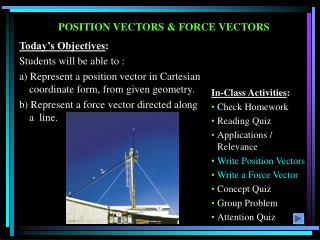DownloadDownload PresentationPOSITION VECTORS & FORCE VECTORS

# POSITION VECTORS & FORCE VECTORS

Télécharger la présentation## POSITION VECTORS & FORCE VECTORS

- - - - - - - - - - - - - - - - - - - - - - - - - - - E N D - - - - - - - - - - - - - - - - - - - - - - - - - - -
##### Presentation Transcript

1. Today’s Objectives: Students will be able to : a) Represent a position vector in Cartesian coordinate form, from given geometry. b) Represent a force vector directed along a line. POSITION VECTORS & FORCE VECTORS • In-Class Activities: • Check Homework • Reading Quiz • Applications / Relevance • Write Position Vectors • Write a Force Vector • Concept Quiz • Group Problem • Attention Quiz

2. READING QUIZ 1. A position vector, rPQ, is obtained by A) Coordinates of Q minus coordinates of P B) Coordinates of P minus coordinates of Q C) Coordinates of Q minus coordinates of the origin D) Coordinates of the origin minus coordinates of P 2. A force of magnitude F, directed along a unit vector U, is given by F = ______ . A) F (U) B) U / F C) F / U D) F + U E) F –U

3. This awning is held up by three chains. What are the forces in the chains and how do we find their directions? Why would we want to know these things? APPLICATIONS

4. A position vector is defined as a fixed vector that locates a point in space relative to another point. POSITION VECTOR Consider two points, A and B, in 3-D space. Let their coordinates be (XA, YA, ZA) and (XB, YB, ZB ), respectively.

5. POSITION VECTOR The position vector directed from A to B, r AB , is defined as r AB = {( XB– XA )i + ( YB– YA ) j + ( ZB– ZA ) k }m Please note that B is the ending point and A is the starting point. ALWAYS subtract the “tail” coordinates from the “tip” coordinates!

6. If a force is directed along a line, then we can represent the force vector in Cartesian coordinates by using a unit vector and the force’s magnitude. So we need to: FORCE VECTOR DIRECTED ALONG A LINE (Section 2.8) a) Find the position vector, rAB, along two points on that line. b) Find the unit vector describing the line’s direction, uAB = (rAB/rAB). c) Multiply the unit vector by the magnitude of the force, F = F uAB .

7. EXAMPLE Given: The 420 N force along the cable AC. Find: The force FAC in the Cartesian vector form. Plan: 1. Find the position vector rAC and the unit vector uAC. 2. Obtain the force vector as FAC = 420 N uAC .

8. EXAMPLE (continued) As per the figure, when relating A to C, we will have to go 2 m in the x-direction, 3 m in the y-direction, and -6 m in the z-direction. Hence, rAC = {2 i+ 3 j 6 k} m. (We can also find rAC by subtracting the coordinates of A from the coordinates of C.) rAC = (22 + 32 + 62)1/2 = 7 m Now uAC= rAC/rAC and FAC = 420 uAC N = 420 (rAC/rAC ) So FAC = 420{ (2 i+ 3 j 6 k) / 7 } N = {120 i + 180 j - 360 k } N

9. CONCEPT QUIZ 1. P and Q are two points in a 3-D space. How are the position vectors rPQ and rQP related? A) rPQ = rQP B) rPQ = -rQP C) rPQ = 1/rQP D) rPQ = 2 rQP 2. If F and r are force vector and position vectors, respectively, in SI units, what are the units of the expression (r * (F / F)) ? A) Newton B) Dimensionless C) Meter D) Newton - Meter E) The expression is algebraically illegal.

10. Given: Two forces are acting on a pipe as shown in the figure. Find: The magnitude and the coordinate direction angles of the resultant force. GROUP PROBLEM SOLVING Plan: 1) Find the forces along CA and CB in the Cartesian vector form. 2) Add the two forces to get the resultant force, FR. 3) Determine the magnitude and the coordinate angles of FR.

11. FCA= 100 lb (rCA/rCA) FCA = 100 lb (–3 sin 40°i + 3 cos 40°j – 4 k)/5 FCA= (– 38.57 i + 45.96 j – 80 k) lb GROUP PROBLEM SOLVING (continued) FCB = 81 lb (rCB/rCB) FCB = 81 lb (4 i – 7 j – 4 k)/9 FCB= {36i– 63 j – 36 k} lb

12. GROUP PROBLEM SOLVING (continued) FR = FCA + FCB = {– 2.57 i – 17.04 j – 116 k} lb FR = (2.572 + 17.042 + 1162) = 117.3 lb = 117 lb  = cos-1(–2.57/117.3) = 91.3°  = cos-1(–17.04/117.3) = 98.4°  = cos-1(–116/117.3) = 172°

13. ATTENTION QUIZ 1. Two points in 3 – D space have coordinates of P (1, 2, 3) and Q (4, 5, 6) meters. The position vector rQP is given by A) {3 i + 3 j + 3 k}m B) {– 3 i– 3 j– 3 k}m C) {5 i + 7 j + 9 k} m D) {– 3 i + 3j+ 3 k} m E) {4 i + 5 j + 6 k} m 2. Force vector, F, directed along a line PQ is given by A) (F/ F) rPQ B) rPQ/rPQ C) F(rPQ/rPQ) D) F(rPQ/rPQ)

14. End of the Lecture Let Learning Continue